## Sample Test Problems

1. We wish to get a good upper limit on the Helium ground state energy. Use as a trial wave function the 1s hydrogen state with the parameter a screened nuclear charge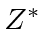to get this limit. Determine the value ofwhich gives the best limit. The integralfor a nucleus of charge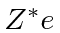.
2. A Helium atom has two electrons bound to anucleus. We have to add the coulomb repulsion term (between the two electrons) to the zeroth order Hamiltonian.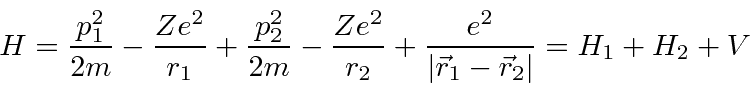The first excited state of Helium has one electron in the 1S state and the other in the 2S state. Calculate the energy of this state to zeroth order in the perturbation V. Give the answer in eV. The spins of the two electrons can be added to give states of total spin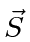. The possible total spin states areand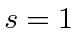. Write out the full first excited Helium state which hasand. Include the spatial wave function and don't forget the Pauli principle. Use bra-ket notation to calculate the energy shift to this state in first order perturbation theory. Don't do any integrals.

Jim Branson 2013-04-22